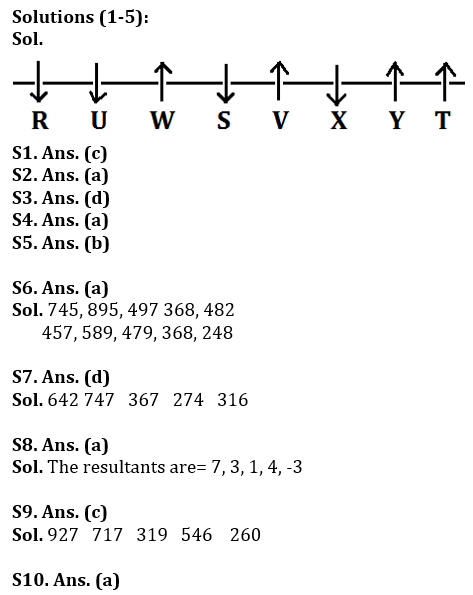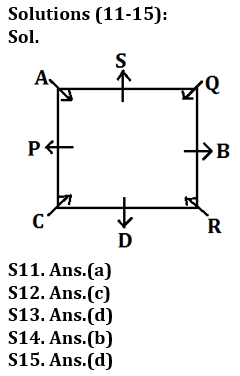# Bank of Baroda AO Reasoning Quiz 2023 – 7 March

Topic: Seating arrangements, series

Directions (1-5): Study the following information carefully and answer the questions given below.

Eight friends are sitting in a straight line. Some of them are facing north and some are facing south. R, who sits at the end, sits third to S’s right. is second to the right of YV. Only three persons sit between W and Y, Y does not sit at the end of the row. V is sitting third to the left of U, who is W’s immediate neighbour. V does not face south. sits second to the left of TX, which sits third to the right of W. R sits to the left of T and faces the opposite direction of T. Y is in north direction.

Q1. Who among the following exactly fits between W and Y?

(a) S

(b) R

(c) V

(d) X

(e) T

Q2. Who among the following is sitting third to the left of Y?

(a) S

(b) T

(c) U

(d) V

(E) W

Q3. Form a group such that four of the following five are similar in a particular way, which of the following does not belong to that group?

(a) R

(b) S

(c) X

(d) Y

(E) U

Q4. How many persons fit between Y and U?

(a) Four

(b) Three

(c) None

(d) Two

(e) one

Q5. Who sits fourth to the right of the one sitting immediately to the right of V?

(a) W

(b) U

(c) X

(d) T

(e) Y

Directions (6-10): The following questions are based on three five-digit numbers given below:

634 784 386 257 371

Q6. If 1 is added to all the digits of each number and arranged in ascending order, what will be the second lowest number after the arrangement?

(a) 257
(b) 784
(c) 634
(d) 371
(e) None of these

Q7. If the second digit is subtracted from one, it is replaced by the third digit in each number, which number is the highest after this arrangement?

(a) 257
(b) 371
(c) 386
(d) 784

(e) None of these

Q8. If the first and third digits of each number are added and then the second digit is subtracted, how many numbers greater than 5 will there be after this process?

(a) One

(b) Two

(c) Three

(d) Four

(e) None of these

Q9. If in each number, subtract 1 from all odd digits and add 3 to each even digit, which of the following numbers will be smallest after this process?

(a) 634
(b) 386
(c) 371
(d) 784
(e) None of these

Q10. If all the digits of a given number are arranged in descending order, which of the following numbers will be the largest?

(a) 784
(b) 634
(c) 257
(d) 386
(e) None of these

Directions (11-15): Study the following information carefully and answer the questions given below:

Eight persons are seated around a square table in such a way that four of them sit at the four corners and the others sit in the middle of the four sides. The persons sitting in the corners face the center and the persons sitting in the middle face outwards.

A third sits to the right of AD. B sits on the other side to the left of the one sitting opposite to S. Q sits to the left of the one immediately to the right of R. Sits opposite the AR. Sits in front of PB. C does not fit in the middle of the sides.

Q11. Who exactly sits between P and B as measured from the right of P?

(a) S

(b) Q

(c)

(d) a

(e) R

Q12. How many persons fit between D and Q when counted from the left of Q?

(a) Four

(b) Three

(c) Two

(d) one

(e) None

Q13. Who among the following sits immediately to the right of P?

(a) R

(b) C

(c) Q

(d) a

(e)B

Q14. Form a group such that four of the following five are similar in a particular way, which of the following does not belong to that group?

(a) S

(b) Q

(c) P

(d) d

(e)B

Q15. Who among the following sits third to the left of the one sitting to the right of Q?

(a) S

(b) C

(c) R

(d)B

(E) P

Solution: